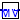## Buildings.Fluid.Sensors.Conversions

Package with conversions for sensor models

### Information

This package contains models to convert sensor output.

Extends from Modelica.Icons.Package (Icon for standard packages).

### Package Content

Name DescriptionTo_VolumeFraction Conversion from mass fraction to volume fractionExamples Collection of models that illustrate model use and test models

##Buildings.Fluid.Sensors.Conversions.To_VolumeFraction

Conversion from mass fraction to volume fraction### Information

This component converts mass fraction to volume fraction for an ideal gas. The default value for the parameter `MMBul` assumes that the bulk medium is air. The model assumes that the concentration of the measured substance is small enough to be neglected in the molar mass of the mixture.

### Parameters

TypeNameDefaultDescription
MolarMassMMMea Molar mass of measured substance [kg/mol]
MolarMassMMBulModelica.Media.IdealGases.Co...Molar mass of bulk medium [kg/mol]

### Connectors

TypeNameDescription
input RealInputmMass fraction
output RealOutputVVolume fraction

### Modelica definition

model To_VolumeFraction "Conversion from mass fraction to volume fraction" parameter Modelica.SIunits.MolarMass MMMea "Molar mass of measured substance"; parameter Modelica.SIunits.MolarMass MMBul=Modelica.Media.IdealGases.Common.SingleGasesData.Air.MM "Molar mass of bulk medium"; Modelica.Blocks.Interfaces.RealInput m "Mass fraction"; Modelica.Blocks.Interfaces.RealOutput V "Volume fraction"; protected parameter Real con = MMBul/MMMea "Conversion coefficient from mass to volume fraction"; equation V = con * m; end To_VolumeFraction;